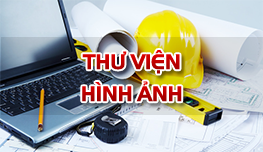Tài liệu học tập

### Mini test 4 (Thủy lực)

• Đăng bởi: nguyenphuocbinh
• Sinh viên
• 18/01/2019

Test 4

1. The standard atmospheric pressure is 101.32 kPA, The local atmospheric pressure at a location was 91.52 kPA, If a pressure is recorded as 22.48 kPa (gauge), it is equivalent to :

A, 123.80 kPa (abs)

B, 88.84 kPa (abs)

C, 114.00 kPa (abs)

D, 69.04 kPa (abs)

1. A U-tube manometermeasures :

A, absolute pressure at a point

B, local atmospheric pressure

C, difference in total energy between two points

D, difference in pressure between two points

1. In the setup shown in the following figure assuming the specific weight of water as 10 kN/m3, the pressure difference between the two points A and B will be :

A, 100 Pa

B, -100 Pa

C, 200 Pa

D, -200 Pa

1. An inclined manometercontains a liquid of relative density 0.8 and has an inclination of 30oto the horizontal. For a certain pressure, the column length was 10 cm. If there is an uncertainty of 1o in the measurement of the angle of inclination, the calculated pressure would have an uncertainty of :

A, 1 %

B, 0.28 %

C, 1.75 %

D, 3 %

1. A U-tube open at both ends and made of 8 mm diameter glass tube has mercury in the bottom to a height of 10 cm above the horizontal limB, If 19 cm of water is added to one of the limbs, the difference in mercury levelsat equilibrium is :

A, 3.0 cm

B, 2.8 cm

C, 1.0 cm

D, Zero

1. For a submergedplanein a liquid, the resultant hydrostatic force on one side of the plane is related to area A, centroid depth h, depth of the center of pressure hcp and depth of bottom edge hb as F is:

A, γAhcp

B, γAh

C, γAhb

D, Ah/γ

1. For an inclined plane submerged in a liquid, the center of fluid pressureon one side of the plane will be :

A, above the top edge of the area

B, vertically below the center of gravity

C, below the center of gravity

D, in the same horizontal plane as the center of gravity

1. An equilateral triangular plateis immersed in water as shown in the following figure, The center of pressurebelow the water surface is at a depth of :

A, 3h/4

B, h/3

C, 2h/3

D, h/2

1. hen the water surface coincides with the top edge of a rectangulargate 2.5 m wide × 3 m deep, the depth of center of pressureis :

A, 1.0 m

B, 1.5 m

C, 2.0 m

D, 2.5 m

1. A circularplate of diameter Dis submerged in water vertically so that the top most point is just at the water surface. The center of pressure of the plate will be below the water surface at a depth of :

A, 5D/8

B, 11D/16

C, 2D/3

D, 3D/4